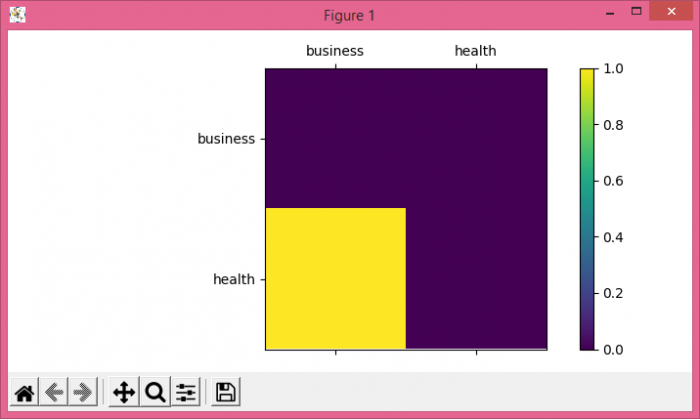# How to plot a confusion matrix with string axis rather than integer in Python?

To plot a confusion matrix with string axis rather than integer in Python, we can take the following steps−

• Make a list for labels.
• Create a confusion matrix. Use confusion_matrix() to calculate accuracy of classification.
• 3. Add an '~.axes.Axes' to the figure as part of a subplot arrangement.
• Plot the values of a 2D matrix or array as a color-coded image.
• Using colorbar() method, create a colorbar for a ScalarMappable instance, *mappable*
• 6. Set x and y ticklabels using set_xticklabels and set_yticklabels methods.
• To display the figure, use show() method.

## Example

from matplotlib import pyplot as plt
from sklearn.metrics import confusion_matrix
plt.rcParams["figure.figsize"] = [7.00, 3.50]
plt.rcParams["figure.autolayout"] = True
cm = confusion_matrix(, )
fig = plt.figure()
plt.show()